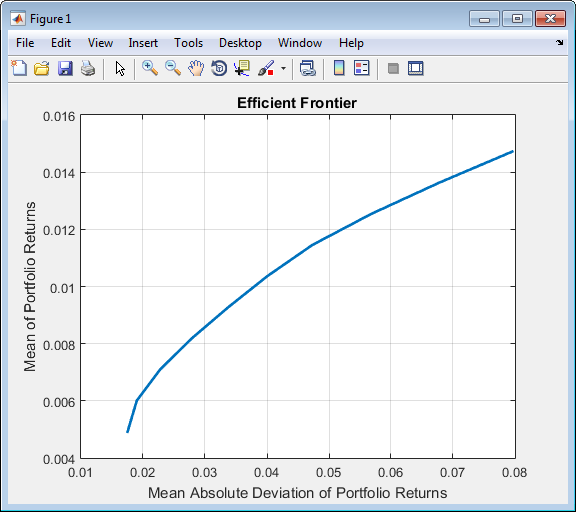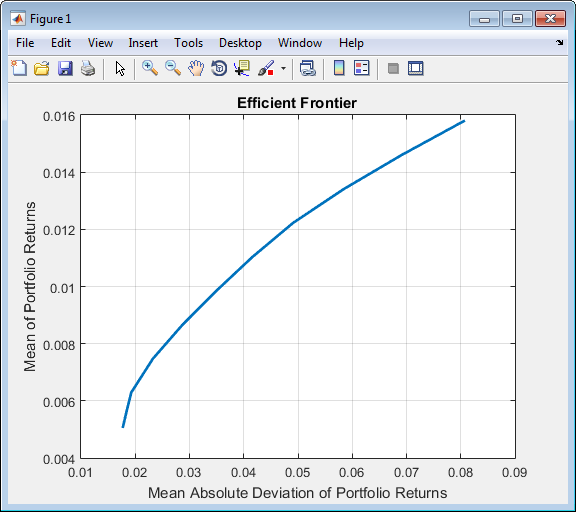Documentation

Creating the PortfolioMAD Object

To create a fully specified MAD portfolio optimization problem, instantiate the PortfolioMAD object using PortfolioMAD. For information on the workflow when using PortfolioMAD objects, see PortfolioMAD Object Workflow.

Syntax

Use PortfolioMAD to create an instance of an object of the PortfolioMAD class. You can use the PortfolioMAD object in several ways. To set up a portfolio optimization problem in a PortfolioMAD object, the simplest syntax is:

This syntax creates a PortfolioMAD object, p, such that all object properties are empty.

The PortfolioMAD object also accepts collections of argument name-value pair arguments for properties and their values. The PortfolioMAD object accepts inputs for public properties with the general syntax:

p = PortfolioMAD('property1', value1, 'property2', value2, ... );

If a PortfolioMAD object already exists, the syntax permits the first (and only the first argument) of PortfolioMAD to be an existing object with subsequent argument name-value pair arguments for properties to be added or modified. For example, given an existing PortfolioMAD object in p, the general syntax is:

p = PortfolioMAD(p, 'property1', value1, 'property2', value2, ... );

Input argument names are not case-sensitive, but must be completely specified. In addition, several properties can be specified with alternative argument names (see Shortcuts for Property Names). The PortfolioMAD object tries to detect problem dimensions from the inputs and, once set, subsequent inputs can undergo various scalar or matrix expansion operations that simplify the overall process to formulate a problem. In addition, a PortfolioMAD object is a value object so that, given portfolio p, the following code creates two objects, p and q, that are distinct:

q = PortfolioMAD(p, ...)

A MAD portfolio optimization problem is completely specified with the PortfolioMAD object if the following three conditions are met:

• You must specify a collection of asset returns or prices known as scenarios such that all scenarios are finite asset returns or prices. These scenarios are meant to be samples from the underlying probability distribution of asset returns. This condition can be satisfied by the setScenarios function or with several canned scenario simulation functions.

• The set of feasible portfolios must be a nonempty compact set, where a compact set is closed and bounded. You can satisfy this condition using an extensive collection of properties that define different types of constraints to form a set of feasible portfolios. Since such sets must be bounded, either explicit or implicit constraints can be imposed and several tools, such as the estimateBounds function, provide ways to ensure that your problem is properly formulated.

Although the general sufficient conditions for MAD portfolio optimization go beyond these conditions, the PortfolioMAD object handles all these additional conditions.

If you create a PortfolioMAD object, p, with no input arguments, you can display it using disp:

disp(p);

SellCost: []
RiskFreeRate: []
Turnover: []
SellTurnover: []
NumScenarios: []
Name: []
NumAssets: []
AssetList: []
InitPort: []
AInequality: []
bInequality: []
AEquality: []
bEquality: []
LowerBound: []
UpperBound: []
LowerBudget: []
UpperBudget: []
GroupMatrix: []
LowerGroup: []
UpperGroup: []
GroupA: []
GroupB: []
LowerRatio: []
UpperRatio: []

The approaches listed provide a way to set up a portfolio optimization problem with the PortfolioMAD object. The custom set functions offer additional ways to set and modify collections of properties in the PortfolioMAD object.

Using the PortfolioMAD Function for a Single-Step Setup

You can use the PortfolioMAD object to directly set up a “standard” portfolio optimization problem. Given scenarios of asset returns in the variable AssetScenarios, this problem is completely specified as follows:

m = [ 0.05; 0.1; 0.12; 0.18 ];
C = [ 0.0064 0.00408 0.00192 0;
0.00408 0.0289 0.0204 0.0119;
0.00192 0.0204 0.0576 0.0336;
0 0.0119 0.0336 0.1225 ];
m = m/12;
C = C/12;

AssetScenarios = mvnrnd(m, C, 20000);

p = PortfolioMAD('Scenarios', AssetScenarios, ...
'LowerBound', 0, 'LowerBudget', 1, 'UpperBudget', 1);
The LowerBound property value undergoes scalar expansion since AssetScenarios provides the dimensions of the problem.

You can use dot notation with the function plotFrontier.

p.plotFrontier;Using the PortfolioMAD Function with a Sequence of Steps

An alternative way to accomplish the same task of setting up a “standard” MAD portfolio optimization problem, given AssetScenarios variable is:

m = [ 0.05; 0.1; 0.12; 0.18 ];
C = [ 0.0064 0.00408 0.00192 0;
0.00408 0.0289 0.0204 0.0119;
0.00192 0.0204 0.0576 0.0336;
0 0.0119 0.0336 0.1225 ];

m = m/12;
C = C/12;

AssetScenarios = mvnrnd(m, C, 20000);

p = setScenarios(p, AssetScenarios);
p = PortfolioMAD(p, 'LowerBound', 0);
p = PortfolioMAD(p, 'LowerBudget', 1, 'UpperBudget', 1);

plotFrontier(p);This way works because the calls to the PortfolioMAD object are in this particular order. In this case, the call to initialize AssetScenarios provides the dimensions for the problem. If you were to do this step last, you would have to explicitly dimension the LowerBound property as follows:

m = [ 0.05; 0.1; 0.12; 0.18 ];
C = [ 0.0064 0.00408 0.00192 0;
0.00408 0.0289 0.0204 0.0119;
0.00192 0.0204 0.0576 0.0336;
0 0.0119 0.0336 0.1225 ];

m = m/12;
C = C/12;

AssetScenarios = mvnrnd(m, C, 20000);

p = PortfolioMAD(p, 'LowerBound', zeros(size(m)));
p = PortfolioMAD(p, 'LowerBudget', 1, 'UpperBudget', 1);
p = setScenarios(p, AssetScenarios);

Note

If you did not specify the size of LowerBound but, instead, input a scalar argument, the PortfolioMAD object assumes that you are defining a single-asset problem and produces an error at the call to set asset scenarios with four assets.

Shortcuts for Property Names

The PortfolioMAD object has shorter argument names that replace longer argument names associated with specific properties of the PortfolioMAD object. For example, rather than enter 'AInequality', the PortfolioMAD object accepts the case-insensitive name 'ai' to set the AInequality property in a PortfolioMAD object. Every shorter argument name corresponds with a single property in the PortfolioMAD object. The one exception is the alternative argument name 'budget', which signifies both the LowerBudget and UpperBudget properties. When 'budget' is used, then the LowerBudget and UpperBudget properties are set to the same value to form an equality budget constraint.

Shortcuts for Property Names

Shortcut Argument Name

Equivalent Argument / Property Name

ae

AEquality

ai

AInequality

assetnames or assets

AssetList

be

bEquality

bi

bInequality

budget

UpperBudget and LowerBudget

group

GroupMatrix

lb

LowerBound

n or num

NumAssets

rfr

RiskFreeRate

scenario or assetscenarios

Scenarios

ub

UpperBound

For example, this call to PortfolioMAD uses these shortcuts for properties:

m = [ 0.05; 0.1; 0.12; 0.18 ];
C = [ 0.0064 0.00408 0.00192 0;
0.00408 0.0289 0.0204 0.0119;
0.00192 0.0204 0.0576 0.0336;
0 0.0119 0.0336 0.1225 ];

m = m/12;
C = C/12;

AssetScenarios = mvnrnd(m, C, 20000);

p = PortfolioMAD('scenario', AssetScenarios, 'lb', 0, 'budget', 1);
plotFrontier(p);

Direct Setting of Portfolio Object Properties

Although not recommended, you can set properties directly using dot notation, however no error-checking is done on your inputs:

m = [ 0.05; 0.1; 0.12; 0.18 ];
C = [ 0.0064 0.00408 0.00192 0;
0.00408 0.0289 0.0204 0.0119;
0.00192 0.0204 0.0576 0.0336;
0 0.0119 0.0336 0.1225 ];
m = m/12;
C = C/12;

AssetScenarios = mvnrnd(m, C, 20000);

p = setScenarios(p, AssetScenarios);

p.LowerBudget = 1;
p.UpperBudget = 1;
p.LowerBound = zeros(size(m));

plotFrontier(p);

Note

Scenarios cannot be assigned directly using dot notation to a PortfolioMAD object. Scenarios must always be set through either the PortfolioMAD object, the setScenarios function, or any of the scenario simulation functions.